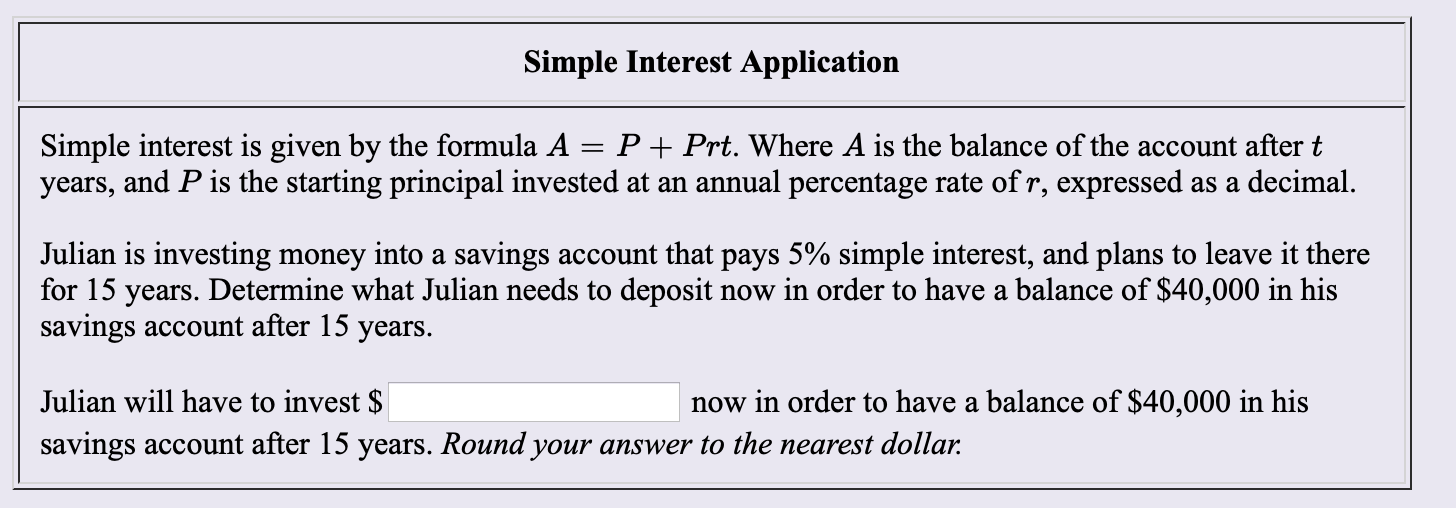# Simple Interest Application PPrt. Where A is the balance of the account after t Simple interest is given by the formula A years, and P is the starting principal invested at an annual percentage rate of r, expressed as a decimal. Julian is investing money into a savings account that pays 5% simple interest, and plans to leave it there for 15 years. Determine what Julian needs to deposit savings account after 15 years. now in order to have a balance of $40,000 in his Julian will have to invest$ now in order to have a balance of $40,000 in his savings account after 15 years. Round your answer to the nearest dollar. Questionhelp_outlineImage TranscriptioncloseSimple Interest Application PPrt. Where A is the balance of the account after t Simple interest is given by the formula A years, and P is the starting principal invested at an annual percentage rate of r, expressed as a decimal. Julian is investing money into a savings account that pays 5% simple interest, and plans to leave it there for 15 years. Determine what Julian needs to deposit savings account after 15 years. now in order to have a balance of$40,000 in his Julian will have to invest $now in order to have a balance of$40,000 in his savings account after 15 years. Round your answer to the nearest dollar. fullscreen

### Want to see the step-by-step answer?